Function Repository Resource:

# BisectList

Make a pair of lists consisting of alternate terms from the original list

Contributed by: George Beck
 ResourceFunction["BisectList"][{a1,a2,a3,a4,a5,a6,…}] makes the list {{a1,a3,a5…},{a2,a4,a6,…}}.

## Details and Options

ResourceFunction["BisectList"] is a partial inverse function of Riffle.
The two sublists have equal lengths.
If the input list has an odd number of terms, the last term is ignored.
The argument can be an expression other than a list.

## Examples

### Basic Examples (3)

The lists being riffled have the same length:

 In:=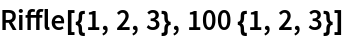Out=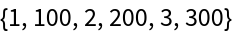BisectList gives back the sublists:

 In:=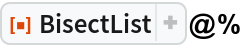Out=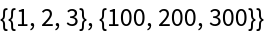Here, the riffled lists have different lengths:

 In:=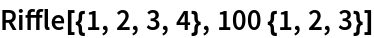Out=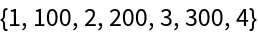BisectList ignores the last term:

 In:=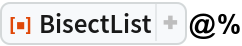Out=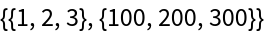Here the head of the expression is not List:

 In:=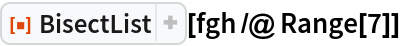Out=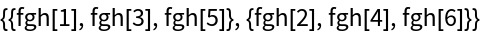### Scope (3)

Add consecutive pairs of a list:

 In:=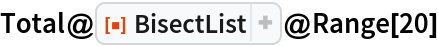Out=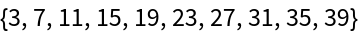Apply BisectList twice:

 In:=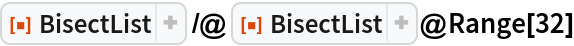Out=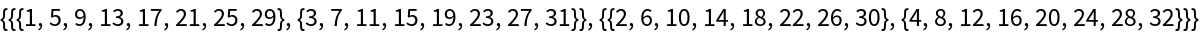Pull out the interleaved subsequences, making them both positive:

 In:=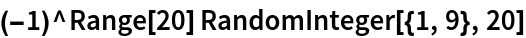Out=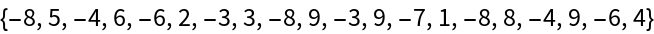In:=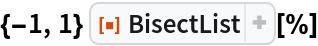Out=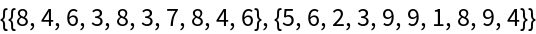George Beck

## Requirements

Wolfram Language 11.3 (March 2018) or above

## Version History

• 1.0.0 – 01 February 2019